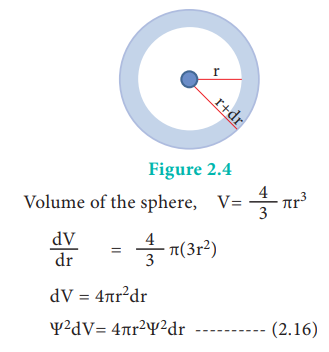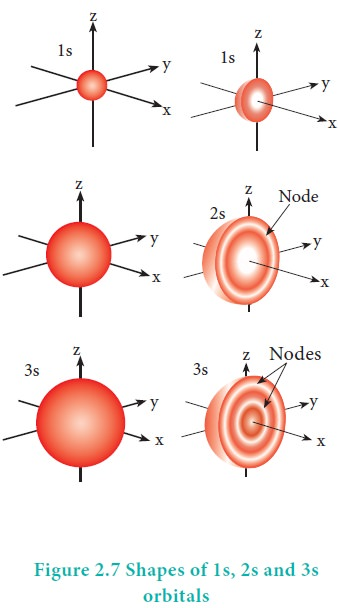Home | | Chemistry 11th std | Quantum numbers

# Quantum numbers

The electron in an atom can be characterised by a set of four quantum numbers, namely principal quantum number (n), azimuthal quantum number (l), magnetic quantum number (m) and spin quantum number (s).

Quantum numbers

The electron in an atom can be characterised by a set of four quantum numbers, namely principal quantum number (n), azimuthal quantum number (l), magnetic quantum number (m) and spin quantum number (s). When Schr├Čdinger equation is solved for a wave function ╬©, the solution contains the first three quantum numbers n, l and m. The fourth quantum number arises due to the spinning of the electron about its own axis. However, classical pictures of species spinning around themselves are incorrect.

### Principal quantum number (n):

This quantum number represents the energy level in which electron revolves around the nucleus and is denoted by the symbol 'n'.

1. The 'n' can have the values 1, 2, 3,ŌĆ” n=1 represents K shell; n=2 represents L shell and n = 3, 4, 5 represent the M, N, O shells, respectively.

2. The maximum number of electrons that can be accommodated in a given shell is 2n2.

3. 'n' gives the energy of the electron, En= (-1312.8)Z2/n2 kJ mol-1 1 and the distance of the electron from the nucleus is given by rn=(0.529)n2/Z A

### Azimuthal Quantum number (l) or subsidiary quantum number :

1. It is represented by the letter 'l', and can take integral values from zero to n-1, where n is the principal quantum number

2. Each l value represents a subshell (orbital). l = 0, 1, 2, 3 and 4 represents the s, p, d, f and g orbitals respectively.

3. The maximum number of electrons that can be accommodated in a given subshell (orbital) is 2(2l+1).

4. It is used to calculate the orbital angular momentum using the expression### Magnetic quantum number (ml):

1. It is denoted by the letter 'ml'. It takes integral values ranging from -l to +l through 0. i.e. if l=1; m = -1, 0 and +1

2. Different values of m for a given l value, represent different orientation of orbitals in space.

3. The Zeeman Effect (the splitting of spectral lines in a magnetic field) provides the experimental justification for this quantum number.

4. The magnitude of the angular momentum is determined by the quantum number l while its direction is given by magnetic quantum number.

### Spin quantum number (ms):

1. The spin quantum number represents the spin of the electron and is denoted by the letter 'ms'

2. The electron in an atom revolves not only around the nucleus but also spins. It is usual to write this as electron spins about its own axis either in a clockwise direction or in anti-clockwise direction. The visualisation is not true. However spin is to be understood as representing a property that revealed itself in magnetic fields.

3. Corresponding to the clockwise and anti-clockwise spinning of the electron, maximum two values are possible for this quantum number.

4. The values of 'ms' is equal to -┬Į and +┬Į

### Table 2.1 Quantum numbers and its significanceThe labels on the orbitals, such as px, dz2, fxyz etc. are not associated with specific 'm' values

## Shapes of atomic orbitals:

The solution to Schr├¢dinger equation gives the permitted energy values called eigen values and the wave functions corresponding to the eigen values are called atomic orbitals. The solution (╬©) of the Schr├¢dinger wave equation for one electron system like hydrogen can be represented in the following form in spherical polar coordinates r, ╬Ė, Žå as,

╬© (r, ╬Ė, Žå) = R(r).f(╬Ė).g(Žå) ------                                                                                      (2.15)

(where R(r) is called radial wave function, other two functions are called angular wave functions)

As we know, the ╬© itself has no physical meaning and the square of the wave function |╬©|2 is related to the probability of finding the electrons within a given volume of space. Let us analyse how |╬©|2 varies with the distance from nucleus (radial distribution of the probability) and the direction from the nucleus (angular distribution of the probability).

Consider a single electron of hydrogen atom in the ground state for which the quantum numbers are n=1 and l=0. i.e. it occupies 1s orbital. The plot of R(r)2 versus r for 1s orbital is given in Figure 2.3The graph shows that as the distance between the electron and the nucleus decreases, the probability of finding the electron increases. At r=0, the quantity R(r)2 is maximum i.e. The maximum value for |╬©|2 is at the nucleus. However, probability of finding the electron in a given spherical shell around the nucleus is important. Let us consider the volume (dV) bounded by two spheres of radii r and r+dr.The plot of 4ŽĆr2╬©2 versus r is given below.The above plot shows that the maximum probability occurs at distance of 0.52 ├ģ from the nucleus. This is equal to the Bohr radius. It indicates that the maximum probability of finding the electron around the nucleus is at this distance. However, there is a probability to find the electron at other distances also. The radial distribution function of 2s, 3s, 3p and 3d orbitals of the hydrogen atom are represented as follows.For 2s orbital, as the distance from nucleus r increases, the probability density first increases, reaches a small maximum followed by a sharp decrease to zero and then increases to another maximum, after that decreases to zero. The region where this probability density function reduces to zero is called nodal surface or a radial node. In general, it has been found that ns-orbital has (nŌĆō1) nodes. In other words, number of radial nodes for 2s orbital is one, for 3s orbital it is two and so on. The plot of 4ŽĆr2Žł2 versus r for 3p and 3d orbitals shows similar pattern but the number of radial nodes are equal to(n-l-1) (where n is principal quantum number and l is azimuthal quantum number of the orbital).

### Angular distribution function:

The variation of the probability of locating the electron on a sphere with nucleus at its centre depends on the azimuthal quantum number of the orbital in which the electron is present. For 1s orbital, l=0 and m=0. f(╬Ė) = 1/ŌłÜ2 and g(Žå) = 1/ŌłÜ2ŽĆ. Therefore, the angular distribution function is equal to 1/2ŌłÜŽĆ. i.e. it is independent of the angle ╬Ė and Hence, the probability of finding the electron is independent of the direction from the nucleus. The shape of the s orbital is spherical as shown in the figure 2.7For p orbitals l=1 and the corresponding m values are -1, 0 and +1. The angular distribution functions are quite complex and are not discussed here. The shape of the p orbital is shown in Figure 2.8. The three different m values indicates that there are three different orientations possible for p orbitals. These orbitals are designated as px, py and pz and the angular distribution for these orbitals shows that the lobes are along the x, y and z axis respectively. As seen in the Figure 2.8 the 2p orbitals have one nodal plane.For ŌĆśdŌĆÖ  orbital  l =  2   and  the corresponding m values are -2, -1, 0 +1,+2. The shape of the d orbital looks like a 'clover leaf '. The five m values give rise to five d orbitals namely dxy , dyz, dzx, dx2, dz2, and dz2. The 3d orbitals contain two nodal planes as shown in Figure 2.9.For 'f ' orbital, l = 3 and the m values are -3, -2,-1, 0, +1, +2, +3 corresponding to seven f orbitals fz3, fxz2, fyz2, fxyz, fz(x2ŌłÆy2), fx(x2ŌłÆ3y2), fy(3x2ŌłÆy2), which are shown in Figure 2.10. There are 3 nodal planes in the f-orbitals.## Energies of orbitals

In hydrogen atom, only one electron is present. For such one electron system, the energy of the electron in the nth orbit is given by the expressionFrom this equation, we know that the energy depends only on the value of principal quantum number. As the n value increases the energy of the orbital also increases. The energies of various orbitals will be in the following order:

1s < 2s = 2p < 3s = 3p = 3d <4s = 4p = 4d = 4f < 5s = 5p = 5d = 5f < 6s = 6p = 6d = 6f < 7s

The electron in the hydrogen atom occupies the 1s orbital that has the lowest energy. This state is called ground state. When this electron gains some energy, it moves to the higher energy orbitals such as 2s, 2p etcŌĆ” These states are called excited states.

However, the above order is not true for atoms other than hydrogen (multi-electron systems). For such systems the Schr├Čdinger equation is quite complex. For these systems the relative order of energies of various orbitals is given approximately by the (n+l) rule. It states that, the lower the value of (n + l) for an orbital, the lower is its energy. If two orbitals have the same value of (n + l), the orbital with lower value of n will have the lower energy. Using this rule the order of energies of various orbitals can be expressed as follows.Based on the (n+l) rule, the increasing order of energies of orbitals is as follows:

1s < 2s < 2p < 3s < 3p < 4s <3d <4p <5s<4d < 5p < 6s<4f< 5d < 6p < 7s < 5f < 6d

As we know there are three different orientations in space that are possible for a p orbital. All the three p orbitals, namely, px, py and pz have same energies and are called degenerate orbitals. However, in the presence of magnetic or electric field the degeneracy is lost.

In a multi-electron atom, in addition to the electrostatic attractive force between the electron and nucleus, there exists a repulsive force among the electrons. These two forces are operating in the opposite direction. This results in the decrease in the nuclear force of attraction on electron. The net charge experienced by the electron is called effective nuclear charge. The effective nuclear charge depends on the shape of the orbitals and it decreases with increase in azimuthal quantum number l. The order of the effective nuclear charge felt by a electron in an orbital within the given shell is s > p > d > f. Greater the effective nuclear charge, greater is the stability of the orbital. Hence, within a given energy level, the energy of the orbitals are in the following order. s < p < d < f.The energies of same orbital decrease with an increase in the atomic number. For example, the energy of the 2s orbital of hydrogen atom is greater than that of 2s orbital of lithium and that of lithium is greater than that of sodium and so on, that is, E2s(H) > E2s(Li) > E2s(Na) > E2s(K).

Study Material, Lecturing Notes, Assignment, Reference, Wiki description explanation, brief detail
11th Chemistry : UNIT 2 : Quantum Mechanical Model of Atom : Quantum numbers |